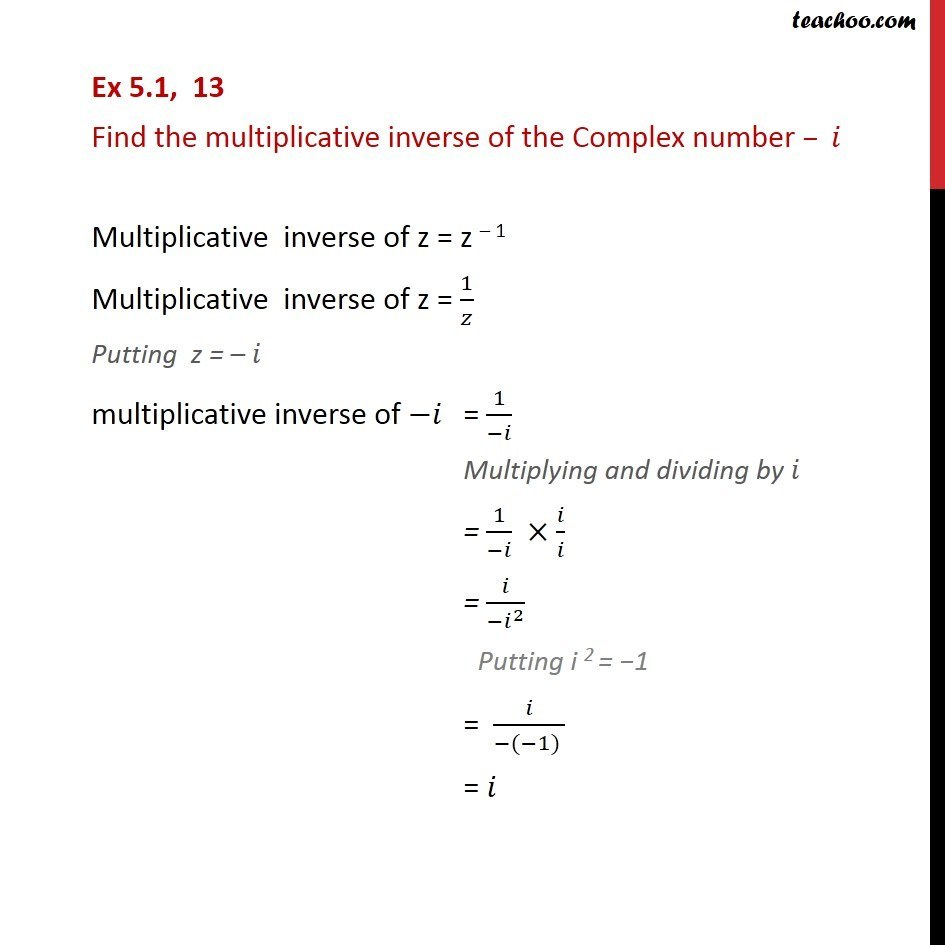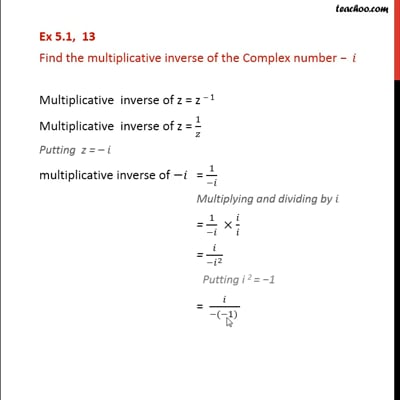Ex 5.1

Chapter 5 Class 11 Complex Numbers (Term 1)
Serial order wiseThis video is only available for Teachoo black users

### Transcript

Ex 5.1, 13 Find the multiplicative inverse of the Complex number Multiplicative inverse of z = z 1 Multiplicative inverse of z = 1/ Putting z = multiplicative inverse of = 1/( ) Multiplying and dividing by = 1/( ) / = /( ^2 ) Putting i 2 = 1 = /( ( 1) ) =